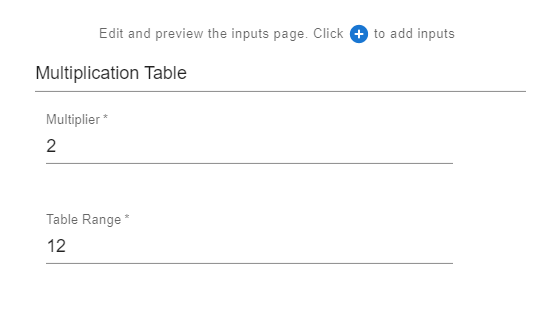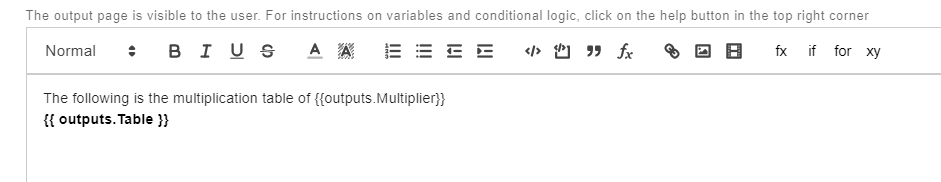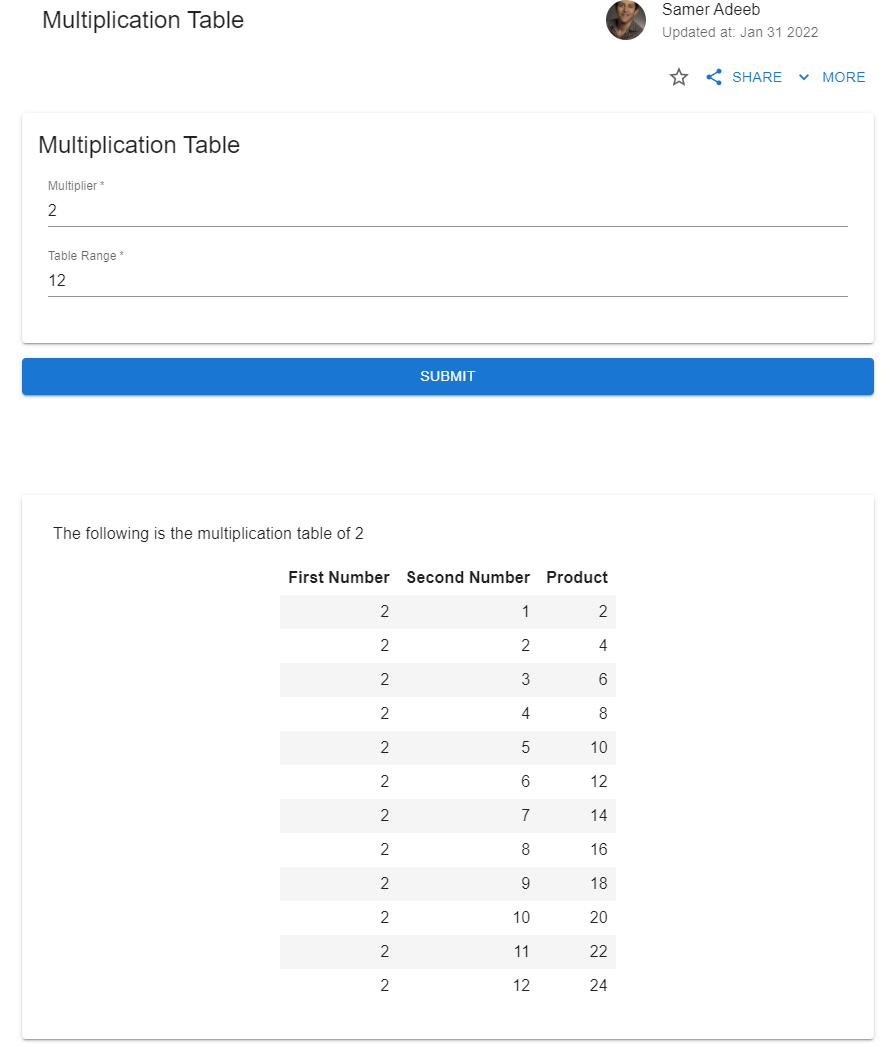# Example 3: Generating a Multiplication Table

This tutorial provides two ways to create an output table by either using a utility function or the Pandas library.

Here is a demo using the utility function:

## Step 1: Info​

• You can fill in the required fields as shown in the following image.
• You can use the default Favicon Image.
• The Primary Image used is available here.## Step 2: Inputs​

For this example, create one input group titled "Multiplication Table" with two numerical inputs: "Multiplier" and "Table Range". The variable names assigned to these inputs are multiplier and range. The following image shows the inputs step after creating these inputs:The following two images show the settings used for each of the inputs:## Step 3: Code​

We are going to present two different versions of the code that can be used to generate tables.

In the first version, the utility function build_html_table, which has the following inputs and outputs:

• columns: an array of table column values.
• ColumnHeaders: an array of strings containing the column headers.
• Returns: a string representing the HTML version of the table.
def build_html_table(columns, ColumnHeaders):    # <table> is the table    # <tr> is table row    # <th> is table header    # <td> is table data cell    # (1) Table opening table    html_table = "<table border='3' cellpadding='5' style='border-collapse:collapse;'><tr>"    # (2) Add header row    rows=len(columns)    for h in ColumnHeaders:        html_table += "<th>"+h+"</th>"    html_table += "</tr>"    # (3) Add all data rows    for i in range(rows):        html_table += "<tr>"        for column in columns:            html_table += "<td>"+str(column[i])+"</td>"        html_table += "</tr>"    # (4) Add the table closing tag    html_table += "</table>"    return html_tabledef main(inputs):    m = inputs['multiplier']    r = inputs['range']    col1 = [m for i in range(r)]    col2 = [i+1 for i in range(r)]    col3 = [col1[i]*col2[i] for i in range(r)]    columns = [col1,col2,col3]    ColumnHeaders = ["First Number", "Second Number","Product"]    table = build_html_table(columns,ColumnHeaders)    return {"Multiplier": m, "Table":table }

In the second version, we are going to use the Pandas library to first create a Pandas dataframe, and then convert the dataframe into an HTML table, using .to_html():

import pandas as pddef main(inputs):    m = inputs['multiplier']    r = inputs['range']    rows = [[m,i+1,m*(i+1)] for i in range(r)]    ColumnHeaders = ["First Number", "Second Number","Product"]    a = pd.DataFrame(data=rows, columns=ColumnHeaders)    table = a.to_html(index=False)    return {"Multiplier": m, "Table":table }

Both versions return an HTML table that can be embedded directly into the output page as shown in the next step.

## Step 4: Output​

The output page used for this example is shown in the following image:## Step 5: Docs​

You can skip adding documentation for this tutorial.

## Step 6: Preview​

In the preview section, confirm that the app works as expected:Once ready, click on PUBLISH to publish the app on the web. The two versions of the app are available here:

https://www.mecsimcalc.com/app/5389912/multiplication_table

https://www.mecsimcalc.com/app/6325826/multiplication_table